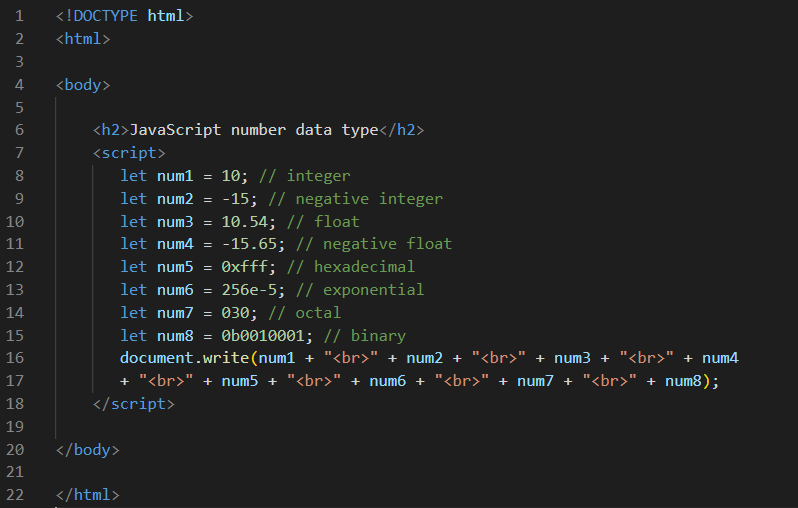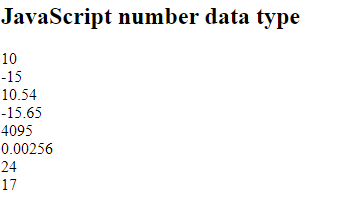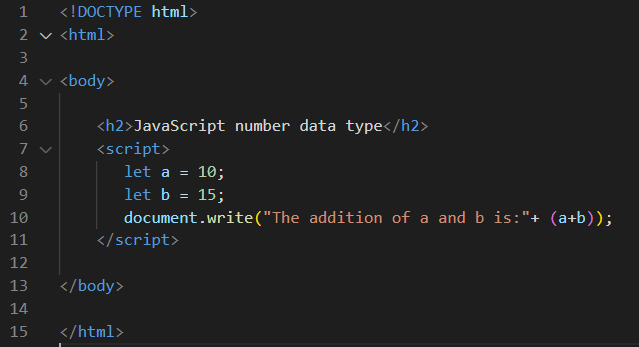JavaScript number data type

# JavaScript number data type

JavaScript number data type
Numbers values that can be processed and calculated-either positive(e.g.,12) or negative(e.g. -12), and floating point(e.g.,23.4).
Example1: Program for print NumberOutputExample2: Program for addition of two numbersOutput